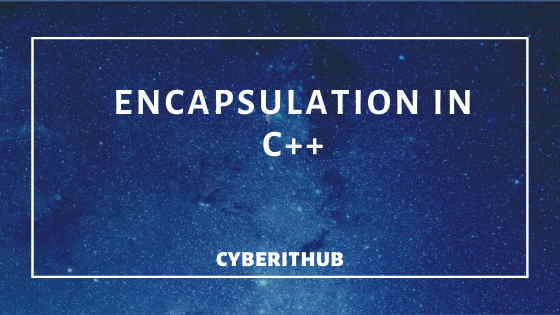# C++(v17) Tutorial: Concepts of Encapsulation with Best Example

In this tutorial, I will take you through the concepts of Encapsulation in C++. It was designed with a bias toward system programming and embedded, resource-constrained software and large systems, with performance, efficiency¸ and flexibility of use as its design highlights.

C++ has also been found useful in many other contexts, with key strengths being software infrastructure and resource-constrained applications,including desktop applications, servers (e.g. e-commerce, Web search, or SQL servers), and performance-critical applications (e.g. telephone switches or space probes)

## Concepts of Encapsulation

Encapsulation is one of the feature of OOPs concept which helps in binding together the data and functions that manipulates those data.### ENCAPSULATION

It led to the concept of data hiding. The concepts of encapsulation is often used to hide the internal representation, or state, of an object from the outside .Example would be a simple class which is considered as user defined data type. Encapsulation binds multiple data and data functions in a single unit called class.

### Example

```#include<iostream>
using namespace std;
class Parent{
int x;
public:
void setX(int a){x=a;}
int getX() {return x;}
};

int main()
{
Parent b;
b.setX(10);
cout<<"Value for x is:"<<"\t"<<b.getX();
return 0;
}
```

### Output

`Value for x is: 10`
So we have encapsulated data x and functions setX(), getX() in a single class called Base.

### Program not an Encapsulation

```#include<iostream>
using namespace std;
class Parent{
public:
int x;
};

int main()
{
Parent b;
b.x =10;
cout<< "Value for x is:"<<"\t"<<b.x;
return 0;
}```

### Output

`Value for x is: 10`
Here only data is defined inside the class which is getting manipulated by the outsider function(not part of class Base).Such cases are not example of encapsulation.

### ABSTRACTION

Abstraction is also one of the important feature of OOPs concept using which we only expose the interfaces and the hide the implementation detail from the users. Real life example would be Refrigerator. We increase/decrease the temperature of refrigerator using the button without actually knowing how it is implemented in the background. Hence user only interact with the interface(button here) and doesn't know it's implementation(logic behind them control using that button).

### Difference between Encapsulation and Abstraction

There is very minor difference between concepts of encapsulation and abstraction which is ,encapsulation binds the data and functions which manipulate them
in a single unit. Whereas abstraction hides the implementation and expose only the interface to the user.

### Example

```#include<iostream>
using namespace std;
class Refrigerator{
int total;public:
Refrigerator(int i=0){total = i;}
void increaseTemp(int number) {total+= number;} //interface to outside world
void decreaseTemp(int number) {total-= number;} //interface to outside world
};

int main()
{
Refrigerator r;
r.increaseTemp(5);
cout<<"Total"<<"\t"<<r.getTemp()<<endl;
r.decreaseTemp(2);
cout<<"Total"<<"\t"<<r.getTemp();
return 0;
}```

### Output

```Total   5
Total   3   ```